Courses

# Introduction to the Periodic Table - Periodic Properties Chemistry Notes | EduRev

## Chemistry : Introduction to the Periodic Table - Periodic Properties Chemistry Notes | EduRev

The document Introduction to the Periodic Table - Periodic Properties Chemistry Notes | EduRev is a part of the Chemistry Course Inorganic Chemistry.
All you need of Chemistry at this link: Chemistry

PERIODIC PROPERTIES
Periodic Table is the arrangement of the elements in tabular form according to their properties so that similar elements fall within the same vertical column and dissimilar elements are separated.

Periodic Table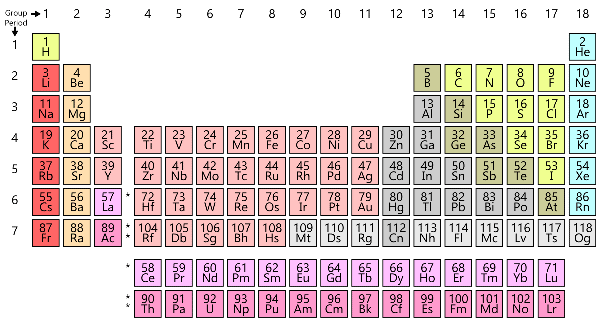Fig: periodic table

MODERN PERIODIC LAW
The properties of elements are periodic functions of their atomic numbers. If elements are arranged in increasing order of their atomic numbers, there is a repetition of properties after 2, 8, 18 and 32 elements.
The numbers 2, 8, 18 and 32 are also referred as magic numbers.

LONG FORM OF PERIODIC TABLE
The elements have been arranged in the increasing order of atomic number.
The horizontal rows constitute periods while the vertical rows constitute groups or families.
Periodic table is divided into four main blocks. These are s, p, and f-blocks. The division of elements into a blocks is primarily based upon their electronic configuration.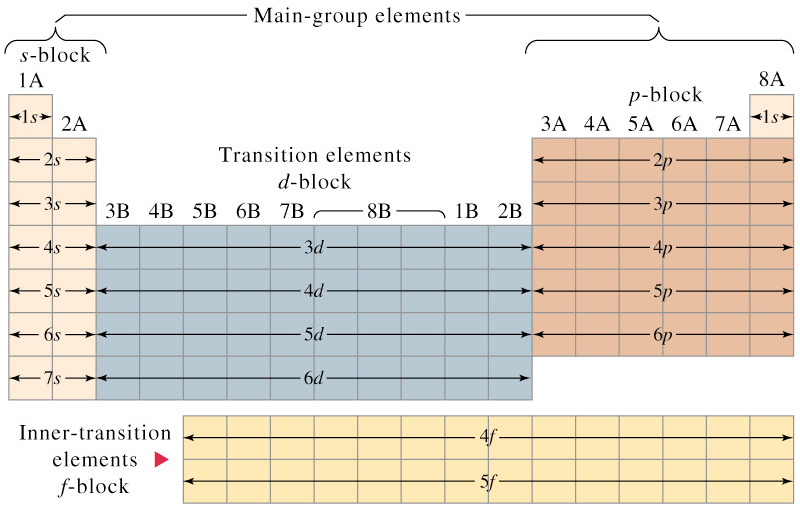Fig: Electronic configuration according to different blocks

Commonly used terms
(i) Representative elements : All the s and p block elements are known as representative elements except zero group.
(ii) Typical elements : Elements of second and third period are known as typical elements.

CLASSIFICATION OF ELEMENTS AS METALS, NON-METALS AND METALLOIDS
(1) Metals: About 80% of the elements are metals. Metals are the elements which are malleable and ductile, possess luster, are good conductors of heat, electricity and have high densities. Metals usually have high melting and boiling points, and are generally solids at room temperature. “Mercury is the only which is liquid at room temperature”. Gallium (303 K) also have very low melting points.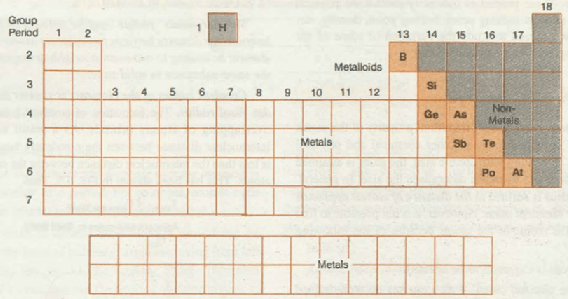Fig: Properties of metals, non-metals and metalloids

(2) Non-Metals: Non-metals are much less in number than metals. Non-metals have low melting and boiling points.
They are usually solids or gases at room temperature. Non-metals are neither malleable nor ductile. They are poor conductors of heat and electricity. The non-metallic character increases going from left to right and metallic character increases going down a group.

(3) Metalloids: There is no sharp dividing metals from non-metals. A zig-zag line separates metals from non-metals as shown in figure. The borderline elements such as silicon, germanium, arsenic, antimony and tellurium exhibit characteristic properties of metals as well as non-metals. These elements are called semimetals or metalloids.

CLASSIFICATION OF ELEMENTS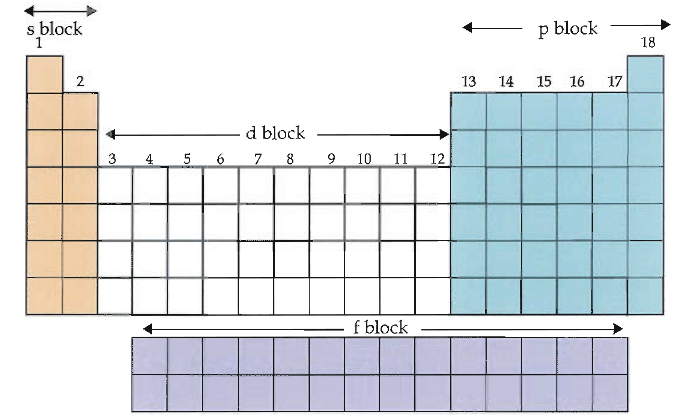On the basis of electronic configuration, the elements may be divided into four groups.
S-block elements

• These are the elements in which last electron enters s subshell.
• These are present in the left part of the periodic table.
• These include group 1 and 2 elements.
• All the s- block elements are metals.
• The general electronic configuration of valence shell for s block elements is ns1-2 ( n = 1 to 7).

P-block elements

• These are the elements in which last electron enters p subshell.
• These are present in the right part of the periodic table.
• These are present in 13 to 18 group of the periodic table.
• Most of the p block elements are metalloids and non metals but some of them are metals also.
• The general electronic configuration of the valence shell is ns2np1-6 ( n = 2 to 7).
ns2 np6 is stable noble gas configuration. The electronic configuration of He is 1s2.

D-Block Elements

• These are the elements in which last electron enters d-subshell.
• These are present in the middle part of the periodic table (between s & p block elements)
• D block elements include group  3 to 12 groups of the periodic table.
• All d block elements are metals.
• The last electrons enters in ( n – 1)d orbital.
• The outermost electronic configuration of d block elements  is (n-1)d1-10 ns1-2 (n = 4 to 7).
• There are three series of d-block elements as under
(i) 3d series – Sc(21) to Zn (30)
(ii) 4d series – Y (39) to Cd (48)
(iii) 5d series – La (57), Hf (72) to Hg (80)

IUPAC NOMENCLATURE FOR ELEMENTS WITH  (Z > 100)
The elements beyond uranium (Z = 92) are all synthetic elements and are known as trans uranium elements. The elements beyond fermium (Z = 100) are known as trans fermium elements. These elements have atomic number 101 onwards.
The elements fermium (Z = 100), mendelevium (Z = 101), nobelium (Z = 102) and lawrencium (Z = 103) are named after the names of famous scientists.

Nomenclature of the elements: The names are derived by using roots for the three digits in the atomic number of the element and adding the ending –ium. The roots for the number are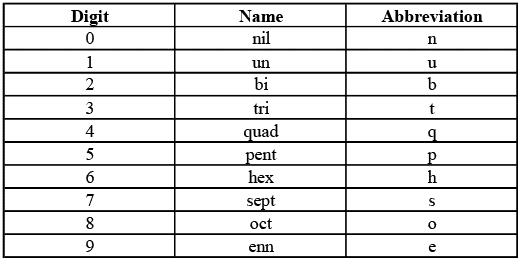Thus element with atomic number 109 will be named as une (u for 1, n for 0 and e for 9).
Table below summarises the names of the elements with atomic number above 100.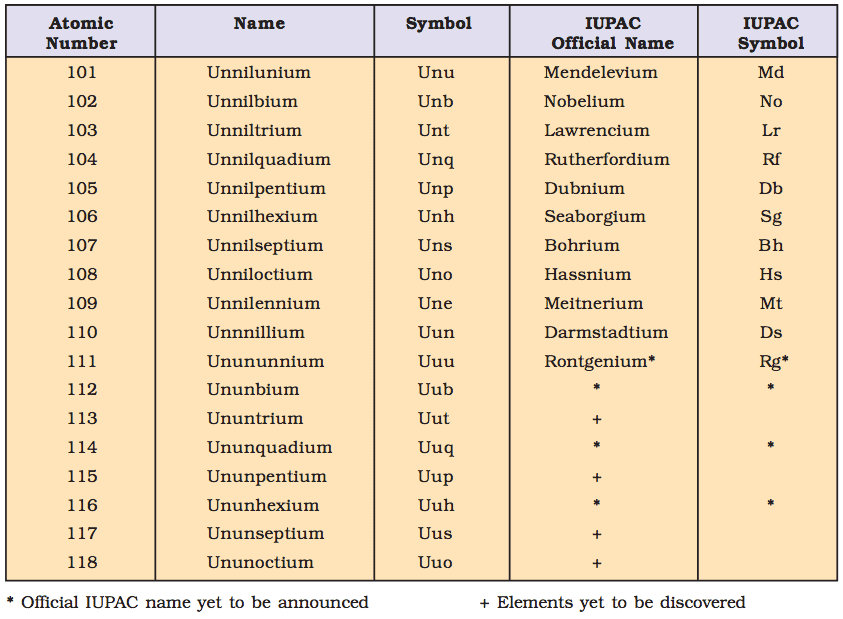EFFECTIVE NUCLEAR CHARGE (Z*)

• It is the nuclear attractive force experienced by the e- when it is shielded by inner lying electrons.
• 2p e is shielded more than or 2s e as it penetrates the 1s orbital less than 2s orbital.
• As a result we have the energy sequence:  [2s < 2p],   [3s < 3p < 3d] and  [4s < 4p < 4d < 4f]
• As we move to atoms of elements of higher atomic number, the energy difference between orbitals of same value of n decreases.

Screening Effect:

• The e-s of inner shell repel the e-s of outer most shells. This effect is known as screening or shielding effect.
• The e-s of outer most shell are thus shielded or screened from the nucleus by inner e-s.
• As a result of screening effect the outer most e-s do not experience the complete nuclear charge.
• So an increase in no. of inner e-s increases screening effect and an increase in screening effect decreases Nuclear charge. This results in decrease of I.E.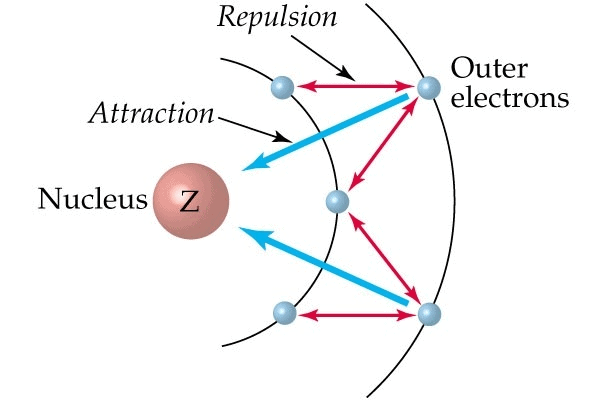Nature of orbitals containing e-s : (Penetrating effect of e-s)

• The penetrating power of e-s towards the nucleus in a given shell decreases in the order s > p > d > f.
• If penetration of e- is more, it will be more tightly held up by the nucleus, so it will be difficult to remove it.
• That means the I.E. increases with increase in penetrating power of e-s in the subshells.

Empirical Rules for estimating ENC -  “Slater Rule”

• The actual nuclear charge experienced by the electrons in different orbitals is estimated by Slater Rules.
• The ENC (Z*) acting on a given e- is obtained by subtracting the screening (shielding) constant ‘S’.

Z*(ENC) = Z(Nuclear charge)S(Shielding constant)
(i) Write the electronic configuration in the following order and groupings (1s), (2s, 2p), (3s, 3p)(3d), (4s, 4p), (4d) (4f), (5s, 5p) etc.
(ii) Electron in any group higher in this sequence than the electron under consideration contribute nothing to ‘S’.
(iii) Then for an e in an ‘ns’ or np orbital.
(a) All other electrons in the (ns, np) group contribute S = 0.35 each.
(b) All electrons in the (n – 1) shell contribute S = 0.85 each.
(c) All electrons in (n – 2) or lower shells contribute S = 1.00 each.
(iv) For an electron in nd or nf orbital, all electrons in the same group contribute s = 0.35 each.
(v) Those in groups lying lower in the sequence than (nd) or (nf) contribute s = 1.00 each.

Example: ‘K’ = (1s2)(2s2p6)(3s2p6)(4s1).
The ENC experienced by ‘4s’ e- is  Z* = Z - S = 19 - (0.85 × 8) + (1.00 × 10) = 2.20
⇒ If we consider the E.C. of K as (1s2)(2s2p6)(3s2p6)(3d1) then Z* would be Z* = 19 - (1 × 18) = 1
⇒Thus the e- in the 4s orbital is under the influence of the greater effective nuclear charge and hence in the ground state it is this orbital that is occupied.

Offer running on EduRev: Apply code STAYHOME200 to get INR 200 off on our premium plan EduRev Infinity!

,

,

,

,

,

,

,

,

,

,

,

,

,

,

,

,

,

,

,

,

,

;